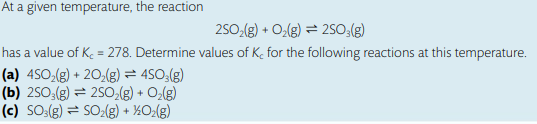# Equilibrium Constant, KcI understand that changing the coefficients of the reaction affects Kc in a certain way;however, I did not know how to do the math part to find the solution. For example, in part ‘‘a’’ I know that I will write the Kc equation by doing the concentration of products over reactants and O2 will be to the power of 2, SO2 to the power of 4 / SO3^4, but I need help in finding how will this change affect the value of Kc and what should I do mathematically to change the number 278 in a correct way.

Hello,

I dont normally answer chemistry questions because I am traumatized from this subject, so please check if you have the answer key after my work.

These questions relate to manipulation of Kc, if you are interested in revising it. The basic and simple idea behind it is that they give you an initial reaction with its Kc then they ask you to derive the Kc for a number of similar other reactions which are all based on the initial one.

Here is the simple table showing how to manipulate Kc and solve all types of questions:Basically, in part a, the reaction was doubled as we can infer from the question where all coefficients in the initial reaction became twice as initially. In the manipulation table, this will make Kc squared, so you square 278.

In part b, the reaction was reversed but the coefficients stayed the same, in this case we will inverse the original Kc, so make it 1/278

In part c, the reaction was reversed AND halved. so we take the square root AND make it 1/(whatever answer you get). i think you can also take the answer of part b and put it under square root.

If this was the answer and you found it helpful, consider marking it as the answer. Otherwise, I am not the best in chem but this is to the best of my abilities

2 Likes